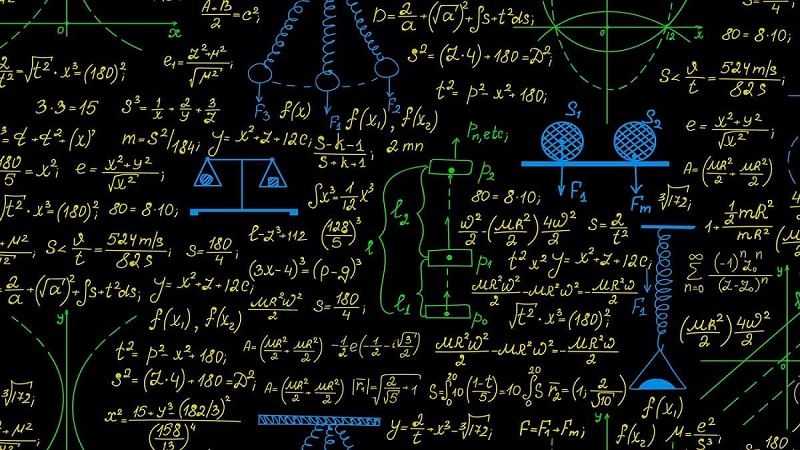# Design and Analysis of Algorithms - CS8451, CS6402

## Online Study Material, Lecturing Notes, Assignment, Reference, Wiki and important questions and answers## Chapter 1 : Introduction

=> Introduction to the Design and Analysis of Algorithms
=> What Is an Algorithm?
=> Fundamentals of Algorithmic Problem Solving
=> Ascertaining the Capabilities of the Computational Device
=> Algorithm Design Techniques
=> Designing an Algorithm and Data Structures
=> Methods of Specifying an Algorithm
=> Proving an Algorithm’s Correctness
=> Analyzing an Algorithm
=> Coding an Algorithm
=> Important Problem Types in Algorithms Analysis
=> Fundamental Data Structures

## Chapter 2 : Fundamentals of the Analysis of Algorithm Eficiency

=> The Analysis Framework
=> Asymptotic Notations and Basic Efficiency Classes
=> Mathematical Analysis of Non recursive Algorithms
=> Mathematical Analysis of Recursive Algorithms
=> Example: Computing the nth Fibonacci Number
=> Empirical Analysis of Algorithms
=> Algorithm Visualization

## Chapter 3 : Brute Force and Exhaustive Search

=> Brute Force and Exhaustive Search
=> Selection Sort and Bubble Sort
=> Sequential Search and Brute-Force String Matching
=> Closest-Pair and Convex-Hull Problems by Brute Force
=> Exhaustive Search
=> Depth-First Search and Breadth-First Search

## Chapter 4 : Decrease and Conquer

=> Decrease and Conquer
=> Insertion Sort
=> Topological Sorting
=> Algorithms for Generating Combinatorial Objects
=> Decrease by a Constant Factor Algorithms
=> Variable Size Decrease Algorithms

## Chapter 5 : Divide and Conquer

=> Divide and Conquer
=> Mergesort
=> Quicksort
=> Binary Tree Traversals and Related Properties
=> Multiplication of Large Integers
=> Strassen’s Matrix Multiplication
=> The Closest Pair Problem by Divide and Conquer
=> Convex Hull Problems by Divide and Conquer

## Chapter 6 : Transform and Conquer

=> Transform and Conquer
=> Presorting
=> Gaussian Elimination
=> Balanced Search Trees: AVL Trees and 2-3 Trees
=> Heaps and Heapsort
=> Horner’s Rule and Binary Exponentiation
=> Problem Reduction

## Chapter 7 : Space and Time Trade Offs

=> Sorting by Counting
=> Input Enhancement in String Matching: Horspool’s and Boyer-Moore Algorithm
=> Open and Closed Hashing
=> B-Trees Algorithms

## Chapter 8 : Dynamic Programming

=> Dynamic Programming
=> Dynamic Programming: Three Basic Examples
=> The Knapsack Problem and Memory Functions
=> Optimal Binary Search Trees
=> Warshall’s and Floyd’s Algorithms

## Chapter 9 : Greedy Technique

=> Greedy Technique
=> Prim’s Algorithm
=> Kruskal’s Algorithm
=> Dijkstra’s Algorithm
=> Huffman Trees and Codes

## Chapter 10 : Iterative Improvement

=> Iterative Improvement
=> The Simplex Method
=> The Iterative Maximum-Flow Problem
=> Maximum Matching in Bipartite Graphs
=> The Stable Marriage Problem

## Chapter 11 : Limitations of Algorithm Power

=> Limitations of Algorithm Power
=> Lower-Bound Arguments
=> Decision Trees algorithms
=> P , NP , and NP-Complete Problems
=> Challenges of Numerical Algorithms

## Chapter 12 : Coping with the Limitations of Algorithm Power

=> Coping with the Limitations of Algorithm Power
=> Backtracking
=> Branch-and-Bound
=> Approximation Algorithms for NP -Hard Problems
=> Approximation Algorithms for the Traveling Salesman Problem
=> Approximation Algorithms for the Knapsack Problem
=> Algorithms for Solving Nonlinear Equations

CS6402 Design and Analysis of Algorithms - Anna University 2013 Regulation Syllabus - Download Pdf
CS8451 Design and Analysis of Algorithms - Anna University 2017 Regulation Syllabus - Download Pdf
Design and Analysis of Algorithms - Question Bank 1 - Download Pdf

Design and Analysis of Algorithms - Question Bank 2 - Download Pdf
Design and Analysis of Algorithms - CS6402 May June 2015 Question Paper
Design and Analysis of Algorithms - CS6402 May June 2016 Question Paper
Design and Analysis of Algorithms - CS6402 May June 2017 Question Paper
Design and Analysis of Algorithms - CS6402 May June 2018 Question Paper
Design and Analysis of Algorithms - CS6402 Nov Dec 2015 Question Paper
Design and Analysis of Algorithms - CS6402 Nov Dec 2016 Question Paper

Design and Analysis of Algorithms - CS6402 Nov Dec 2017 Question Paper